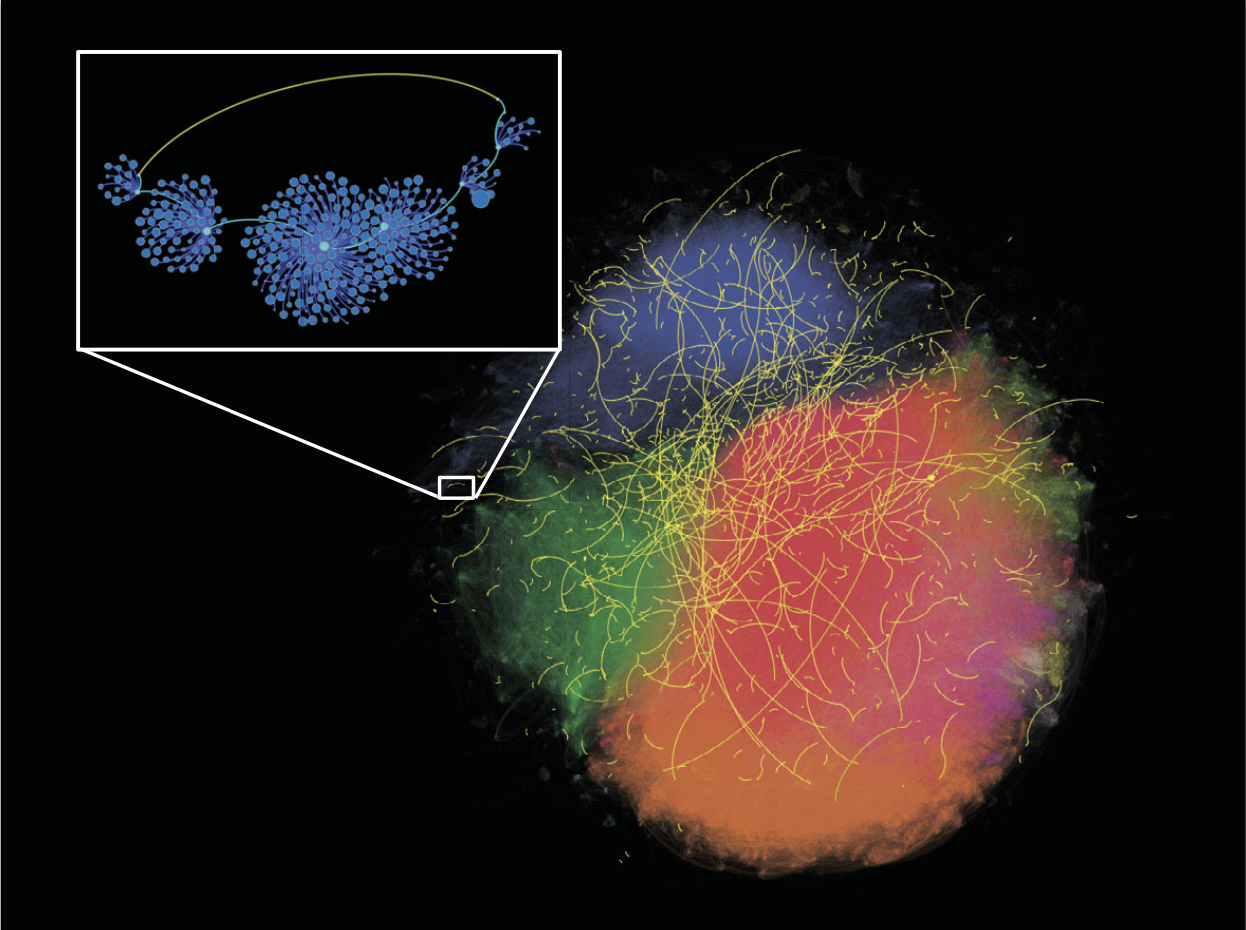# Network WormholesPhoto from Unsplash.

This work was published in Science.

Here’s some basic text.

And here’s some italics

Here’s some bold text.

Here’s a bulleted list:

• First item
• Second item
• Third item

Here’s a numbered list:

1. First
2. Second
3. Third

Python code block:

``````    import numpy as np

def test_function(x, y):
z = np.sum(x,y)
return z
``````

R code block:

``````library(tidyverse)
``````

Here’s some inline code `x+y`.

Here’s an image: <img src=/images/wormholes/singapore_wormholes.png” alt=”Wormholes in the Singapore Twitter Network”> Here’s another image using Kramdown:Here’s some math:

\[z=x+y\]

You can also put it inline \(z=x+y\)

Tags:

Updated: